## ANLYSIS OF THE DYNAMIC BEHAVIOR AND PERFORMANCE OF A VIBRATION ISOLATION SYSTEM WITH GEOMETRIC NONLINEAR FRICTION DAMPING 1)

Liu Xingtian2), Chen Shuhai, Wang Jiadeng, Shen Junfeng

Laboratory of Space Mechanical and Thermal Integrative Technology, Shanghai Institute of Satellite Engineering, Shanghai 201109, China

 基金资助: 国家自然科学基金资助项目.  51505294国家自然科学基金资助项目.  51875363

Received: 2018-09-10   Accepted: 2019-02-26   Online: 2019-03-18

2)刘兴天,高级工程师,主要研究方向：卫星结构设计,结构动力学与振动控制.E-mail:xtliu509@126.comAbstract

In vibration isolation field, nonlinear vibration isolation system catch more attention than linear system because of the better vibration isolation performance. In this paper, a novel nonlinear vibration isolation system with geometric nonlinear friction damping is proposed by add two friction damper that perpendicular to the movement direction of the isolated object. The absolute and relative displacement transmissibility of such kind of vibration isolation system are studied in this paper. Different from the friction damper which usually assuming that the friction force is constant, the friction force studied in this paper is proportional to the displacement of the isolated mass by configuring two linear friction dampers perpendicular to the moving direction of the mass. The mathematical model of the friction damping and the forced vibration of the system are established. The dynamic equation is solved by using Harmonic Balance Method (HBM) subsequently by making some simplification. The result solved by HBM is verified numerically. The performance of the nonlinear vibration isolation system is compared with that of a linear one by the performance index defined by absolute and relative transmissibility. The geometric nonlinear friction can offer small or large friction damping depends on the relative displacement, therefore, the nonlinear friction force can improve the transmissibility for both absolute and relative displacement at resonance and the higher frequencies region if the damping values are chosen carefully which surpass a traditional Kevin vibration isolator model. Meanwhile, the nonlinear vibration isolation system can enlarge the application region for different excitation amplitude and avoid the system failure though the responses of the isolated mass is amplified at low frequency. The vibration isolation system with the configuration of the friction damper proposed is very suitable for both resonance and higher frequencies vibration control. The conclusions given are of importance when design and choosing the friction damping parameters.

Keywords： geometric nonlinear ; isolator ; transmissibility ; friction damping ; passive vibration isolation

Liu Xingtian, Chen Shuhai, Wang Jiadeng, Shen Junfeng. ANLYSIS OF THE DYNAMIC BEHAVIOR AND PERFORMANCE OF A VIBRATION ISOLATION SYSTEM WITH GEOMETRIC NONLINEAR FRICTION DAMPING 1). Chinese Journal of Theoretical and Applied Mechanics[J], 2019, 51(2): 371-379 DOI:10.6052/0459-1879-18-302

## 1 摩擦阻尼和隔振系统建模

### 图 1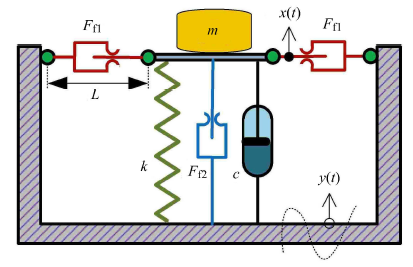Fig.1   Schematic of the vibration isolation system with geometric nonlinear friction damping

$$f_v = 2F_{{\rm f}1} \frac{\left| z \right|\left| \dot {z} \right|^{-1}}{\sqrt {L^2 + z^2} }\dot {z}$$

$$f_v \approx \frac{2F_{f1} }{L}\left| z \right| {\rm sgn} \dot {z}$$

$$m\ddot {z} + c\dot {z} + \left( {F_{{\rm f}2} + \frac{2F_{{\rm f}1} }{L}\left| z \right|} \right) {\rm sgn} \dot {z} + kz = m\omega ^2y_0 \sin \left( {\omega t} \right)$$

$${u}" + 2\zeta {u}' + \left( {\lambda _1 + \lambda _2 \left| u \right|} \right){\rm sgn} {u}' + u = \tilde {y}_0 \Omega ^2\sin (\Omega t)$$

$$u = u_0 \sin \left( {\Omega \tau + \theta } \right)$$

$$-u_0 \Omega ^2\sin \left( {\Omega \tau + \theta } \right) + 2\zeta \Omega u_0 \cos \left( {\Omega \tau + \theta } \right) + \left( {\lambda _1 + \lambda _2 u {\rm sgn} \left( u \right)} \right) {\rm sgn} \left( {u}' \right) + u_0 \sin \left( {\Omega \tau + \theta } \right) = \tilde {y}_0 \Omega ^2\sin (\Omega t)$$

$$Sgn[sin ( \Omega \tau + \theta] \approx \ A_{01} + A_{11} cos ( \Omega \tau + \theta) + A_{21} sin ( \Omega \tau + \theta) \approx \frac{4}{π}sin ( \Omega \tau + \theta)$$

$-u_0 \Omega ^2 + u_0 = \tilde {y}_0 \Omega ^2\cos \theta$

$2\zeta \Omega u_0 + \frac{4}{ π }\lambda _1 + \frac{4}{ π ^2}\lambda _2 u_0 =-\tilde {y}_0 \Omega ^2\sin \theta$

$$( \Omega ^4-2\Omega ^2 + 1 + 4 \zeta ^2 \Omega ^2 + \frac{16}{π^2} \zeta \lambda _2 \Omega + \frac{16 \lambda _2^2 }{ π^4})u_0^2 + \frac{16 \lambda _1^2 }{ π^2} + ( \frac{32}{ π^3} \lambda _1 \lambda _2 + \frac{16}{ π }\zeta \lambda _1 \Omega)u_0-\tilde {y}_0^2 \Omega ^4 = 0$$

$u_0 = \Bigg [-\frac{32}{{π}^3}\lambda _1 \lambda _2 + \qquad \sqrt {\left( {1-\Omega ^2} \right)^2\Bigg( 4\tilde {y}_0^2 \Omega ^4-\frac{64}{{π}^2}\lambda _1^2 \Bigg ) + \frac{64\lambda _2^2 }{{π}^4}\tilde {y}_0^2 \Omega ^4} \Bigg ] \Bigg/ \qquad \Bigg [2\Bigg ( \Omega ^4-2\Omega ^2 + 1 + \frac{16\lambda _2^2 }{{π}^4} \Bigg ) \Bigg ]$

$$T_{\rm a} = \sqrt {u_0^2 + 2y_0 u_0 \cos \theta + y_0^2 } / y_0$$

## 2 仿真与结果

### 图2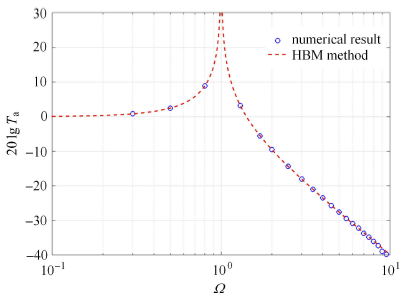Fig.2   Results comparison when$\lambda _1 = 0$,$\lambda _2 = 0$ for HBM and numerical method

### 图3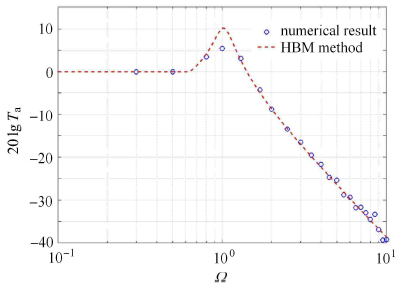Fig.3   Results comparison when$\lambda _1 = 0.3$,$\lambda _2 = 0.5$ for HBM and numerical method

### 图4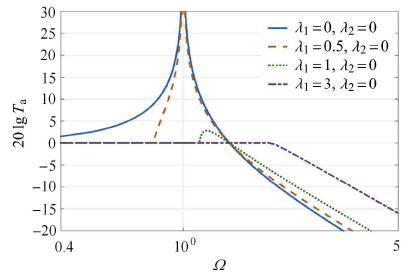Fig.4   Effect of$\lambda _1$ on the absolute transmissibility of the vibration isolation system when$\lambda _2 = 0$

### 图5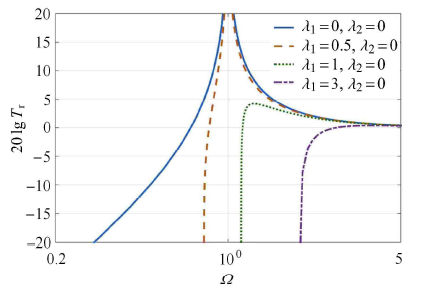Fig.5   Effect of$\lambda _1$ on the relative transmissibility of the vibration isolation system when$\lambda _2 = 0$

### 图6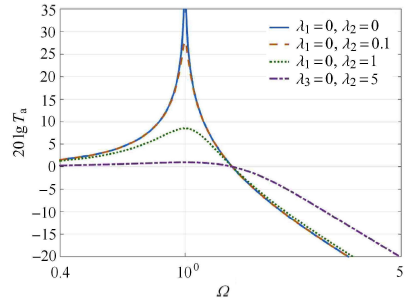Fig.6   Effect of$\lambda _{2}$ on the absolute transmissibility of the vibration isolation system when$\lambda _{1} = 0$

### 图7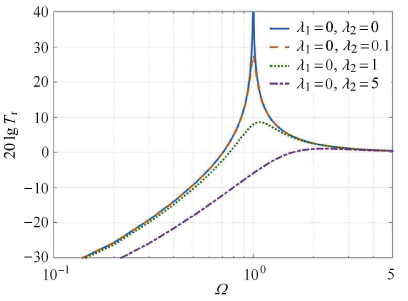Fig.7   Effect of$\lambda _{2}$ on the relative transmissibility of the vibration isolation system when$\lambda _{1} = 0$

### 图8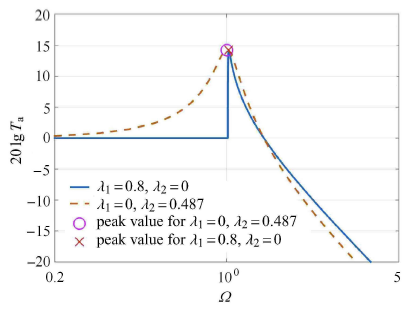Fig.8   Comparison of the absolute transmissibility for the two kinds of vibration isolation system with the same resonance factor

### 图9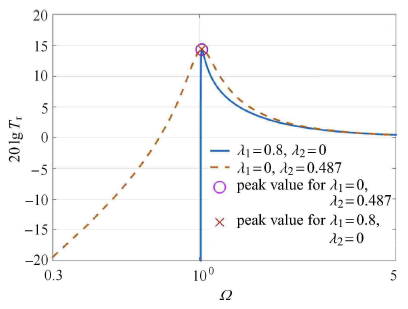Fig.9   Comparison of the relative transmissibility for the two kinds of vibration isolation system with the same resonance factor

### 图10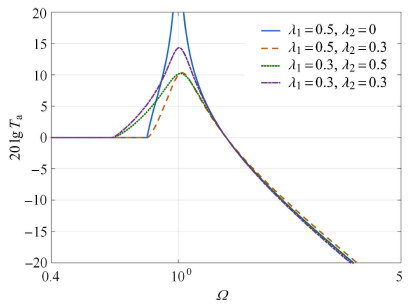Fig.10   Effect of the combination of$\lambda _1$ and$\lambda _2$ on the absolute transmissibility of the nonlinear vibration isolation system

### 图11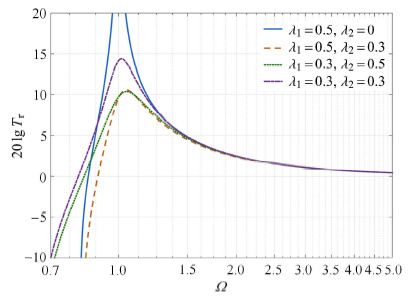Fig.11   Effect of the combination of$\lambda _1$ and$\lambda _2$ on the relative transmissibility of the nonlinear vibration isolation system

### 图12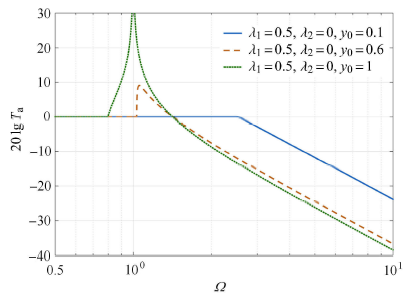Fig.12   Effect of the excitation amplitude on the absolute transmissibility of the constant friction vibration isolation system

### 图13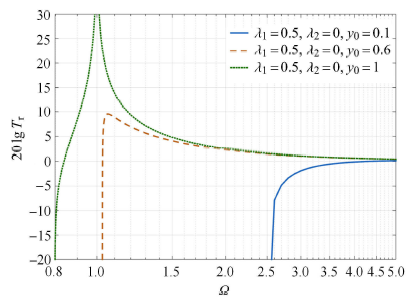Fig.13   Effect of the excitation amplitude on the relative transmissibility of the constant friction vibration isolation system

### 图14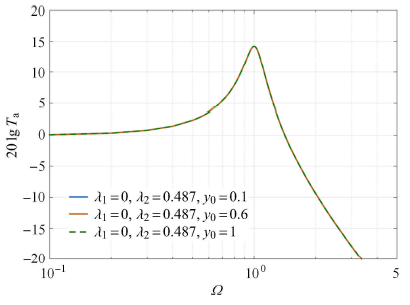Fig.14   Effect of the excitation amplitude on the absolute transmissibility of the nonlinear friction vibration isolation system

### 图15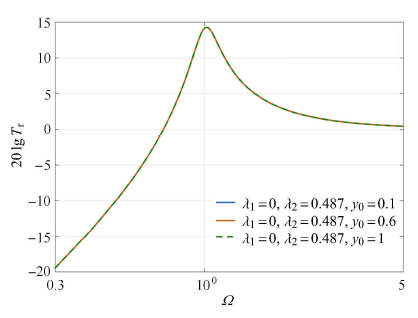Fig.15   Effect of the excitation amplitude on the relative transmissibility of the nonlinear friction vibration isolation system

### 图16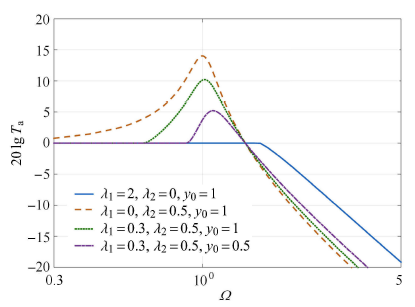Fig.16   Effect of the combination of system parameters on the absolute transmissibility of the vibration isolation system

### 图17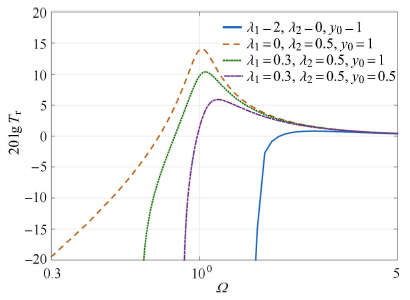Fig.17   Effect of the combination of system parameters on the relative transmissibility of the vibration isolation system

## 3 结 论

(1) 仅就引入几何非线性而言,变阻尼摩擦系数和恒定摩擦系数对绝对传递率和相对传递率的影响类似,即增大变摩擦阻尼系数使系统共振传递率变优而高频衰减变差;

(2) 由于变阻尼摩擦系统的摩擦力和位移相关,因此纯变阻尼摩擦系统无频率"锁定"现象,且该系统能在保持共振峰控制的同时,保持高频衰减;

(3) 与变阻尼摩擦系数相比,在小幅值激励的情况下,恒定摩擦阻尼系统对激励的隔振效果变差,隔振器应用频率范围变小,严重可导致隔振器失效;

(4)通过合理的参数选择,可以使几何非线性系统在共振传递率和高频衰减效果均优于恒定摩擦阻尼系统,同时可以显著避免因激励幅值过小时产生的"锁住"现象,提高系统的可靠性.

The authors have declared that no competing interests exist.

## 参考文献 原文顺序 文献年度倒序 文中引用次数倒序 被引期刊影响因子

Harris C, Piersol A . Shock and Vibration Handbook. New York: McGraw-Hill, 2002

Rivin E . Passive Vibration Isolation. New York: ASME Press, 2003

Ibrahim R .

Recent advances in nonlinear passive vibration isolators

Journal of Sound and Vibration, 2008,314(3-5):371-452

URL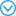The theory of nonlinear vibration isolation has witnessed significant developments due to pressing demands for the protection of structural installations, nuclear reactors, mechanical components, and sensitive instruments from earthquake ground motion, shocks, and impact loads. In view of these demands, engineers and physicists have developed different types of nonlinear vibration isolators. This article presents a comprehensive assessment of recent developments of nonlinear isolators in the absence of active control means. It does not deal with other means of linear or nonlinear vibration absorbers. It begins with the basic concept and features of nonlinear isolators and inherent nonlinear phenomena. Specific types of nonlinear isolators are then discussed, including ultra-low-frequency isolators. For vertical vibration isolation, the treatment of the Euler spring isolator is based on the post-buckling dynamic characteristics of the column elastica and axial stiffness. Exact and approximate analyses of axial stiffness of the post-buckled Euler beam are outlined. Different techniques of reducing the resonant frequency of the isolator are described. Another group is based on the Gospodnetic risch-Fay beam, which is free to slide on two supports. The restoring force of this beam resembles to a great extent the restoring roll moment of biased ships. The base isolation of buildings, bridges, and liquid storage tanks subjected to earthquake ground motion is then described. Base isolation utilizes friction elements, laminated-rubber bearings, and the friction pendulum. Nonlinear viscoelastic and composite material springs, and smart material elements are described in terms of material mechanical characteristics and the dependence of their transmissibility on temperature and excitation amplitude. The article is closed by conclusions, which highlight resolved and unresolved problems and recommendations for future research directions.

URL( Cao Dengqing, Bai Kunchao, Ding Hu , et al.

Advances in dynamics and vibration control of large scale flexible spacecraft

Chinese Journal of Theoretical and Applied Mechanics, 2019,51(1):1-13 (in Chinese))

URLCarrella A, Brennan M, Waters T .

Static analysis of a passive vibration isolator with quasi-zero stiffness characteristic

Journal of Sound and Vibration, 2007,301(3-5):678-689

URLThe frequency range over which a linear passive vibration isolator is effective, is often limited by the mount stiffness required to support a static load. This can be improved upon by employing nonlinear mounts incorporating negative stiffness elements configured in such a way that the dynamic stiffness is much less than the static stiffness. Such nonlinear mounts are used widely in practice, but rigorous analysis, and hence a clear understanding of their behaviour is not readily available in the literature. In this paper, a simple system comprising a vertical spring acting in parallel with two oblique springs is studied. It is shown that there is a unique relationship between the geometry and the stiffness of the springs that yields a system with zero dynamic stiffness at the static equilibrium position. The dynamic stiffness increases monotonically with displacement either side of the equilibrium position, and this is least severe when the oblique springs are inclined at an angle between approximately 48° and 57°. Finally, it is shown that the force–displacement characteristic of the system can be approximated by a cubic equation.

Kovacic I, Brennan M, Waters T .

A study of a nonlinear vibration isolator with a quasi-zero stiffness characteristic

Journal of Sound and Vibration, 2008,315(3):700-711

URLA vibration isolator consisting of a vertical linear spring and two nonlinear pre-stressed oblique springs is considered in this paper. The system has both geometrical and physical nonlinearity. Firstly, a static analysis is carried out. The softening parameter leading to quasi-zero dynamic stiffness at the equilibrium position is obtained as a function of the initial geometry, pre-stress and the stiffness of the springs. The optimal combination of the system parameters is found that maximises the displacement from the equilibrium position when the prescribed stiffness is equal to that of the vertical spring alone. It also satisfies the condition that the dynamic stiffness only changes slightly in the neighbourhood of the static equilibrium position. For these values, a dynamical analysis of the isolator under asymmetric excitation is performed to quantify the undesirable effects of the nonlinearities. It includes considering the possibilities of the appearance of period-doubling bifurcation and its development into chaotic motion. For this purpose, approximate analytical methods and numerical simulations accompanied with qualitative methods including phase plane plots, Poincar maps and Lyapunov exponents are used. Finally, the frequency at which the first period-doubling bifurcation appears is found and the effect of damping on this frequency determined.

Mizuno T, Toumiya T, Takasaki M ,

Vibration isolation system using negative stiffness

Japan Society of Mechanical Engineers International Journal Series C, 2003,46(3):807-812

Zhou N, Liu K .

A tunable high-static-low-dynamic stiffness vibration isolator

Journal of Sound and Vibration, 2010,329(9):1254-1273

URLIn this study, a novel vibration isolator is developed. The developed isolator possesses the characteristics of high-static ow-dynamic stiffness (HSLDS) and can act passively or semi-actively. The HSLDS property of the isolator is obtained by connecting a mechanical spring, in parallel with a magnetic spring that is constructed by a pair of electromagnets and a permanent magnet. The mechanical spring is a structural beam whose stiffness exhibits a hardening behavior. The stiffness of the magnetic spring can be positive or negative, depending on the polarity of the current to the electromagnets. A passive HSLDS isolator is obtained when the electromagnet current is zero. In the stiffness characterization study, the analytical model for each of the springs is established and the tuning parameters are identified. Using the stiffness models, the design optimization issues are investigated. In the experimental study, the effectiveness of the isolator for vibration isolation is tested. The analytical natural frequencies of the isolator are validated experimentally. The relationships between the displacement transmissibility and the exciting frequency are measured both under the passive mode and under the semi-active mode. The on-line tuning capability of the isolator is investigated.

Carrella A, Brennan M, Waters TP , et al.

On the design of a high-static-low-dynamic stiffness isolator using linear mechanical springs and magnets

Journal of Sound and Vibration, 2008,315(3):712-720

URLThe frequency range over which a linear passive vibration isolator is effective is often limited by the mount stiffness required to support a static load. This can be improved upon by incorporating a negative stiffness element in the mount such that the dynamic stiffness is much less than the static stiffness. In this case, it can be referred to as a high-static–low-dynamic stiffness (HSLDS) mount. This paper is concerned with a theoretical and experimental study of one such mount. It comprises two vertical mechanical springs between which an isolated mass is mounted. At the outer edge of each spring, there is a permanent magnet. In the experimental work reported here, the isolated mass is also a magnet arranged so that it is attracted by the other magnets. Thus, the combination of magnets acts as a negative stiffness counteracting the positive stiffness provided by the mechanical springs. Although the HSLDS suspension system will inevitably be nonlinear, it is shown that for small oscillations the mount considered here is linear. The measured transmissibility is compared with a comparable linear mass–spring–damper system to show the advantages offered by the HSLDS mount.

Zhu T, Benjamin C, Robertson W , et al.

Vibration isolation using six degree-of-freedom quasi-zero stiffness magnetic levitation

Journal of Sound and Vibration, 2015,358:48-73

URLIn laboratories and high-tech manufacturing applications, passive vibration isolators are often used to isolate vibration sensitive equipment from ground-borne vibrations. However, in traditional passive isolation devices, where the payload weight is supported by elastic structures with finite stiffness, a design trade-off between the load capacity and the vibration isolation performance is unavoidable. Low stiffness springs are often required to achieve vibration isolation, whilst high stiffness is desired for supporting payload weight. In this paper, a novel design of a six degree of freedom (six-dof) vibration isolator is presented, as well as the control algorithms necessary for stabilising the passively unstable maglev system. The system applies magnetic levitation as the payload support mechanism, which realises inherent quasi-zero stiffness levitation in the vertical direction, and zero stiffness in the other five dofs. While providing near zero stiffness in multiple dofs, the design is also able to generate static magnetic forces to support the payload weight. This negates the trade-off between load capacity and vibration isolation that often exists in traditional isolator designs. The paper firstly presents the novel design concept of the isolator and associated theories, followed by the mechanical and control system designs. Experimental results are then presented to demonstrate the vibration isolation performance of the proposed system in all six directions.

Le T, Kyoung K .

Active pneumatic vibration isolation system using negative stiffness structures for a vehicle seat

Journal of Sound and Vibration, 2014,333(5):1245-1268

URLIn this paper, an active pneumatic vibration isolation system using negative stiffness structures (NSS) for a vehicle seat in low excitation frequencies is proposed, which is named as an active system with NSS. Here, the negative stiffness structures (NSS) are used to minimize the vibratory attraction of a vehicle seat. Owing to the time-varying and nonlinear behavior of the proposed system, it is not easy to build an accurate dynamic for model-based controller design. Thus, an adaptive intelligent backstepping controller (AIBC) is designed to manage the system operation for high-isolation effectiveness. In addition, an auxiliary control effort is also introduced to eliminate the effect of the unpredictable perturbations. Moreover, a radial basis function neural network (RBFNN) model is utilized to estimate the optimal gain of the auxiliary control effort. Final control input and the adaptive law for updating coefficients of the approximate series can be obtained step by step using a suitable Lyapunov function. Afterward, the isolation performance of the proposed system is assessed experimentally. In addition, the effectiveness of the designed controller for the proposed system is also compared with that of the traditional backstepping controller (BC). The experimental results show that the isolation effectiveness of the proposed system is better than that of the active system without NSS. Furthermore, the undesirable chattering phenomenon in control effort is quite reduced by the estimation mechanism. Finally, some concluding remarks are given at the end of the paper.

Sun X, Jing X .

A nonlinear vibration isolator achieving high-static-low-dynamic stiffness and tunable anti-resonance frequency band

Mechanical Systems and Signal Processing, 2016,80:166-188

URL61A 2-D isolator with n-layer Scissor-Like Structure (SLS) is modeled and studied.61The system can achieve good isolation performance in two directions simultaneously.61An excellent anti-resonance frequency band can be achieved with tunable properties.61Experimental results validate the excellent nonlinear stiffness and damping performance.

Sun X, Jing X .

Multi-direction vibration isolation with quasi-zero stiffness by employing geometrical nonlinearity

Mechanical Systems and Signal Processing, 2015, 62-63:149-163

URL61A novel scissor-like structure is employed to construct a three-direction isolator.61The nonlinear stiffness and damping characteristics can be designed via structural parameters.61The system can achieve very excellent quasi-zero stiffness in vibration control in three directions.61The structural nonlinearity is an effective way for beneficial nonlinear stiffness/damping design.

Araki Y, Asai T, Masui T .

Vertical vibration isolator having piecewise-constant restoring force

Earthquake Engineering and Structural Dynamics, 2019,38(13):1505-1523

URL     Magsci<p>分段光滑隔振系统是一类具备分段刚度或阻尼的非线性动力学系统,在振动控制领域中具有广泛代表性,诸如限位隔振系统、分级汽车悬挂等. 分段光滑的刚度或阻尼特性能够实现隔振系统的特定动力学性能及提升隔振性能,如抑制共振响应、提升共振区隔振性能等,但是亦会给隔振系统的动力学行为带来诸多不利影响. 以分段双线性分段光滑隔振系统为理论模型,系统研究了摒除不利于隔振的非线性动力学现象设计方法,包括幅值跳跃、周期运动的倍周期分岔等. 首先,利用平均法与奇异性理论给出了主共振频响曲线拓扑特征的完整拼图. 研究结果表明,参数空间分为4 个区域,其中2 个区域存在幅值跳跃,而其产生跳跃原因分别由鞍结分岔与擦边分岔所导致;基于此提出避免主共振跳跃的设计方法. 其次,建立了隔振有效区内周期运动的庞加莱映射,通过特征值分析给出了避免倍周期分岔发生的条件,证实增大阻尼可以抑制倍周期分岔的发生. 最后通过数值仿真分析了噪声对多稳态运动的影响. 研究结果发现在噪声影响下,分段光滑隔振系统的响应会在不同稳态间跃迁,非常不利于隔振. 因此,在完成跳跃与倍周期分岔的防治设计后,应采用数值仿真校验系统是否存在多稳态运动.</p>

( Gao Xue, Chen Qian, Liu Xianbin .

Nonlinear dynamics design for piecewise smooth vibration isolation system

Chinese Journal of Theoretical and Applied Mechanics, 2016,48(1):192-200 (in Chinese))

URL     Magsci<p>分段光滑隔振系统是一类具备分段刚度或阻尼的非线性动力学系统,在振动控制领域中具有广泛代表性,诸如限位隔振系统、分级汽车悬挂等. 分段光滑的刚度或阻尼特性能够实现隔振系统的特定动力学性能及提升隔振性能,如抑制共振响应、提升共振区隔振性能等,但是亦会给隔振系统的动力学行为带来诸多不利影响. 以分段双线性分段光滑隔振系统为理论模型,系统研究了摒除不利于隔振的非线性动力学现象设计方法,包括幅值跳跃、周期运动的倍周期分岔等. 首先,利用平均法与奇异性理论给出了主共振频响曲线拓扑特征的完整拼图. 研究结果表明,参数空间分为4 个区域,其中2 个区域存在幅值跳跃,而其产生跳跃原因分别由鞍结分岔与擦边分岔所导致;基于此提出避免主共振跳跃的设计方法. 其次,建立了隔振有效区内周期运动的庞加莱映射,通过特征值分析给出了避免倍周期分岔发生的条件,证实增大阻尼可以抑制倍周期分岔的发生. 最后通过数值仿真分析了噪声对多稳态运动的影响. 研究结果发现在噪声影响下,分段光滑隔振系统的响应会在不同稳态间跃迁,非常不利于隔振. 因此,在完成跳跃与倍周期分岔的防治设计后,应采用数值仿真校验系统是否存在多稳态运动.</p>

URL( Lu Zeqi, Chen Liqun .

Some recent progresses in nonlinear passive isolations of vibrations

Chinese Journal of Theoretical and Applied Mechanics, 2017,49(3):550-564 (in Chinese))

URLLu Z, Yang T, Brennan M , et al.

Experimental investigation of a two-stage nonlinear vibration isolation system with high-static-low-dynamic stiffness

ASME Journal of Applied Mechanics, 2017,84(2):021001

Ruzicka J, Derby T . Influence of damping in vibration isolation. Washington, DC: Shock and Vibration Information Center (Defense), 1971

Ravindra B, Mallik A .

Performance of non-linear vibration isolators under harmonic excitation

Journal of Sound and Vibration, 1994,170(3):325-337Vibration isolators having non-linearity in both stiffness and damping terms are analyzed under harmonic excitations. Isolators with symmetric as well as asymmetric restoring forces are considered. The method of harmonic balance is used to obtain the steady state, harmonic response and transmissibility under both force and base excitations. The linear stability analysis of the solutions is presented. A parametric study indicating the effects of various types of damping is reported. The subharmonic and chaotic responses for the systems under investigation will be discussed in a subsequent paper.

Ravindra B, Mallik A .

Hard Duffing-type vibration isolator with combined coulomb and viscous damping

International Journal of Non-linear Mechanics, 1993,28(4):427-440

URLThe steady-state, harmonic response of a vibration isolation system with a cubic, hard non-linear restoring force and combined Coulomb and viscous damping is presented. The results have been obtained by using the method of harmonic balance. It has been assumed that the motion is continuous without any stop. An anomalous jump in the response, similar to the one obtained in earlier studies on certain soft systems, is observed when the isolation system is subjected to a base excitation. Linear stability analysis is carried out to determine the status of the anomalous jump. The effect of the damping parameters on the jump in the response is investigated. Transmissibility curves are plotted for various parameter values to study the performance characteristics. The obtained results extend the previous works of Den Hartog and Ruzicka who considered a linear restoring element.

Thaijaroen W, Harrison A .

Nonlinear dynamic modelling of rubber isolators using six parameters based on parabolic spring, springpot, and smooth-slip friction element

Polymer Testing, 2010,29(7):857-865

URLA time-domain six-parameter model is adopted to simulate the vibration behaviour of rubber isolators over the frequency range of 0.05 25 Hz. The one-dimensional model working under constant preload and temperature is capable of producing force as a function of displacement excitation. The model consists of three components, including a nonlinear parabolic spring, a fractional-derivative-based springpot and a smooth-slip friction element. In order to obtain all required six parameters, a novel standard procedure is proposed based on a two-stage optimization method using two sets of data measured in both amplitude and frequency domains. A number of isolators with differences in shape, composition and mode of deformation are selected for study. The consistency of the proposed optimization method and the accuracy of the obtained model are verified by good agreements between measured and simulated results of stiffness and damping across the ranges of investigated displacement amplitudes and frequencies.

Yang P, Yang J, Ding J .

Dynamic transmissibility of a complex nonlinear coupling isolator

Tsinghua Science and Technology, 2006,11(5):538-542

URLA mathematical model was developed for a complex nonlinear coupling isolator for attenuating vibration which coupled quadratic damping, viscous damping, Coulomb damping, and nonlinear spring forces. The approximate analytical solution for the dynamic transmissibility of the isolator was deduced by combining Fourier transforms and the harmonic balance method with deterministic excitation. The mathematical characteristics of the dynamic transmissibility were analyzed to illustrate the dynamic performance of the isolator. The analytical results show multiple solutions, especially the low-frequency attenuation characteristics below the resonance frequency. The results provide a theoretical basis for the design of nonlinear isolators.

Peng ZK, Lang ZQ, Jing XJ , et al.

The transmissibility of vibration isolators with a nonlinear antisymmetric damping characteristic

Journal of Vibration and Acoustics, 2010,132(1):014501( Peng Zhike, Lang Ziqiang, Meng Guang , et al.

Analysis on transmissibility for a class of nonlinear vibration isolators

Journal of Dynamics and Control, 2011,9(4):314-320 (in Chinese))Peng ZK, Meng G, Lang ZQ , et al.

Study of the effects of cubic nonlinear damping on vibration isolations using Harmonic Balance Method

International Journal of Non-Linear Mechanics, 2012,47(10):1073-1080

URLIn the present study, Harmonic Balance Method (HBM) is applied to investigate the performance of passive vibration isolators with cubic nonlinear damping. The results reveal that introducing either cubic nonlinear damping or linear damping could significantly reduce both the displacement transmissibility and the force transmissibility of the isolators over the resonance region. However, at the non-resonance region where frequency is lower than the resonant frequency, both the linear damping and the cubic nonlinear damping have almost no effect on the isolators. At the non-resonance region with higher frequency, increasing the linear damping has almost no effects on the displacement transmissibility but could raise the force transmissibility. In addition, the influence of the cubic nonlinear damping on the isolators is dependent on the type of the disturbing force. If the strength of the disturbing force is constant and independent of the excitation frequency, then the effect of cubic nonlinear damping on both the force and displacement transmissibility would be negligible. But, when the strength of the disturbing force is dependent of the excitation frequency, increasing the cubic nonlinear damping could slightly reduce the relative displacement transmissibility and increase the absolute displacement transmissibility but could significantly increase the force transmissibility. These conclusions are of significant importance in the analysis and design of nonlinear passive vibration isolators. [All rights reserved Elsevier].

Kovacic I, Milovanovic Z, Brennan M .

On the relative and absolute transmissibility of a vibration isolation system subjected to base excitation

//XXI Conference with International Participation, Noise and Vibration, Serbia, Tara, 2008

URLIn this paper a one-degree of freedom passive vibration isolator system which is subject to harmonic base excitation is analyzed. The isolator is modeled as a parallel combination of a stiffness and damping element with cubic non-linearity. The method of averaging is used to obtain the steady-state harmonic response. A parametric analysis is conducted in order to investigate the influence of the system parameters on the relative and absolute transmissibility of the system from the viewpoint of possible improvement of the transmissibility of a system with linear viscous damping. .

López I, Busturia J, Nijmeijer H .

Energy dissipation of a friction damper

Journal of Sound and Vibration, 2003,278(3):539-561

URLIn this paper the energy dissipated through friction is analysed for a type of friction dampers used to reduce squeal noise from railway wheels. A one degree-of-freedom system is analytically studied. First the existence and stability of a periodic solution are demonstrated and then the energy dissipated per cycle is determined as a function of the system parameters. In this way the influence of the mass, natural frequency and internal damping of the friction damper on the energy dissipation is established. It is shown that increasing the mass and reducing the natural frequency and internal damping of the friction damper maximizes the dissipated energy.

Berger E .

Friction modeling for dynamic system simulation

Applied Mechanics Review, 2002,55(6):535-577

URLThe working of friction modeling tools for dynamic system simulation were discussed. It was observed that in engineering applications, success of models in predicting experimental results depended on the frictional models. It was also observed that the success of frictional model relied on the system dynamic models. Various frictional models from the vast field of engineering models were examined and specific focus was laid on lumped-parameter system models, continuum system models and forced system models.

Stein G, Zahoransky R, Mucka P .

On dry friction modelling and simulation in kinematically excited oscillatory systems

Journal of Sound and Vibration, 2008,311(1-2):74-96

URLThis paper deals with the analysis and simulation of a general single degree of freedom (sdof) oscillatory system with idealised linear viscous damper and dry friction. For dry friction modelling the phenomenological macro-slip approach is employed, described in mathematical form either by the signum function approach or by the physically correct stick lip approach assuming switching phenomena on a short time scale. Both approaches are illustrated first using a steady-state harmonic acceleration excitation with constant amplitude and then a stationary random acceleration excitation, corresponding to a field-measured excitation in a vehicle. The differences in the two approaches are highlighted, indicating that the physically correct stick lip approach describes the friction phenomenon better than the standard signum approach. The signum approach is prone to false numerical oscillations completely distorting the acceleration response signal in comparison to measured suspension system response. The acceleration transmissibility response is analysed in respect to the dry friction force magnitude, employing stationary random excitation. A sdof oscillatory system without viscous damping, subjected to both stationary random acceleration and harmonic acceleration is analysed, too. It is shown that such a system can be used without serious practical problems; however, no implications on its performance from the analysis under harmonic constant amplitude acceleration excitation can be made.

Zhao D, Zhang W, Ma R et al.

Research on a new damper and its application in vibration control of a building

Industrial Construction, 2006,36(2):1-5

URLIt is explored the vibrational energy dissipation theory of a new dry friction damper and its application in vibration control of a building.The research shows that the new dampers have traditional dampers′ merit and overcome the shortcoming that can only offer invariable friction.At the same time,they can be controlled by the signal of the vibration of the building.So the ability of energy dissipation and vibration control effect is augmented.The simulation using finite element program proved that the vibration of the building is reduced with the use of this damper.

Displacement-proportional friction (DPF) in base isolation

Earthquake Engineering and Structural Dynamics, 1987,15(7):799-813

Ferri A .

Friction Damping and Isolation Systems

Journal of Vibration and Acoustics, 1995,117(B):196-206

Ferri A, Whiteman W .

Free response of a system with negative viscous damping and displacement-dependent dry friction damping

Journal of Sound and Vibration, 2007,306(3-5):400-418A stability analysis is conducted of an autonomous single-degree-of-freedom system damped with negative viscous damping and a displacement-dependent Coulomb friction force. The geometry of the dry friction damping element yields a friction force that grows linearly with the system displacement. The most direct application of this system is in the study of a turbomachinery blade with shroud interfaces designed to achieve this geometry. Recent studies have shown that the damping of systems with this type of displacement-dependent dry friction force resembles linear structural damping and suggests that this arrangement may be an effective means of flutter suppression in these turbine and fan blade applications. For this study, the inclusion of negative viscous damping is used in order to approximate destabilizing aerodynamic forces. An exact analysis is conducted to determine the stability of this autonomous system. Results show that energy losses from the displacement-dependent dry friction damper are large enough to achieve local and even global stability under certain conditions.

Whiteman W, Ferri A .

Displacement-dependent dry friction damping of a beam-like structure

Journal of Sound and Vibration, 1996,198(3):313-329

URLThe flexural vibration of a beam-like structure damped with a displacement dependent Coulomb friction force has been examined. Due to the geometry of the dry friction damping element, the friction force grows linearly with the beam's transverse displacement. Harmonic excitation of the system is analyzed using first order harmonic balance and then using an "exact" time domain method. The stick-slip behaviour of the system has also been studied. Even though the only source of damping is dry friction, the system was seen to exhibit damping characteristics similar to linear structural damping. The equivalent natural frequency and equivalent viscous damping were also investigated, and found to depend on the amplitude of response and on the design parameters of the frictional interface. In particular, the overall damping levels as well as the amount of stick-slip motion are seen to depend strongly on the amount of displacement dependence. Another significant result was the appearance of two stable steady-state solutions at certain parameter values. The results suggest that the overall characteristics of mechanical systems may be improved by properly configuring frictional interfaces to allow normal forces to vary with displacement.

Tang B, Brennan M .

A comparison of two nonlinear damping mechanisms in a vibration isolator

Journal of Sound and Vibration, 2013,332(3):510-520

URL78 The influence of two nonlinear dampers on vibration transmissibility are compared. 78 An isolator with geometric nonlinear damping force is studied. 78 Analytical expressions and numerical simulations are used for the analysis. 78 An isolation system with geometrically nonlinear damping has some advantages compared to a linear damper.

/

 〈〉